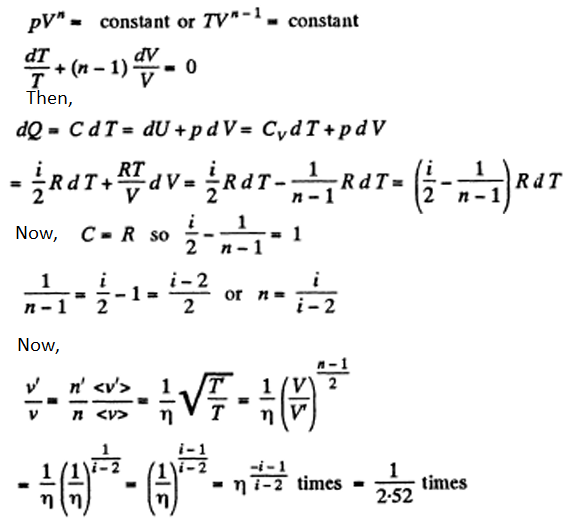# I.E. Irodov Solutions on Kinetic Theory of Gases- Boltzmann's Law and Maxwell's Distribution

Kinetic theory of gases relates the macroscopic property of the gas, like – pressure, temperature, volume to the microscopic property of the gas, like – speed, momentum, position. The gases are made up of a large number of molecules and they are flying in a random direction with a certain speed. The kinetic theory of gases has developed a model that explains the behavior of molecules, which should further explain the behavior of an ideal gas. Expert faculty at BYJU’S prepared solutions for the problems covered in I.E. Irodov book PART TWO. THERMODYNAMICS AND MOLECULAR PHYSICS - 2.3- Kinetic Theory of Gases. Boltzmann's Law and Maxwell's Distribution. By referring to these solutions, students can achieve higher marks in the JEE exam. Students are recommended to go through these solutions thoroughly before the exams to score well and improve their problem-solving abilities.

### I.E. Irodov Solutions on Kinetic Theory of Gases- Boltzmann's Law and Maxwell's Distribution

1. Modern vacuum pumps permit the pressures down to p = 4.10-15 atm to be reached at room temperatures. Assuming that the gas exhausted is nitrogen, find the number of its molecules per 1 cm3 and the mean distance between them at this pressure.

Solution:

1. Using formula, p = nkT

Or n = p/kT = 105 per cc

(Using p = 4 x 10-15 x 1.01 x 105 and k = 1.38 x 10-23 and T = 300)

Mean distance between molecules

(10-5 cc)1/3 = 101/3 x 10-2 cm = 0.2 mm

2. A vessel of volume V = 5.0 l contains m = 1.4 g of nitrogen at a temperature T = 1800 K. Find the gas pressure, taking into account that η = 30% of molecules are disassociated into atoms at this temperature.

Solution:

1. After dissociation each N2 molecule becomes two Adatoms and so contributes, 2 x 3 degrees of freedom. Thus, the number of moles becomes

m/M(1 + η) and p = mRT/MV (1 + η)

M is the molecular weight in grams of N2.

3. Under standard conditions the density of the helium and nitrogen mixture equals p = 0.60 g/l. Find the concentration of helium atoms in the given mixture.

Solution:

1. Let n1 = number density of the atoms

n2 = number density of N2 molecules

Then p = n1 m1 + n2 m2

Where m1 = mass of the atom,

m2 = mass of N2 molecule also p = (n1 + n2) kT

From these two equations we get

$n_1 = \frac{(\frac{p}{kT} - \frac{\rho}{m_2})}{(1-\frac{m_1}{m_2})}$

4. A parallel beam of nitrogen molecules moving with velocity v = 400 m/s impinges on a wall at an angle θ = 30° to its normal. The concentration of molecules in the beam n = 0.9.1019 cm3 . Find the pressure exerted by the beam on the wall assuming the molecules to scatter in accordance with the perfectly elastic collision law.

Solution:

1. $p = \frac{nv \times 2 mv cos \theta \times dA cos \theta}{dA}$

= 2mnv2 cos2 θ

5. How many degrees of freedom have the gas molecules, if under standard conditions the gas density is p = 1.3 mg/cm3 and the velocity of sound propagation in it is v = 330 m/s.

Solution:

1. From the formula

γ = ρV2/p ….(1)

If i = number of degrees of freedom of the gas then

Cp = Cv + RT and Cv = (i/2)RT

γ = Cp/Cv = 1 + 2/i

or i = 2/ (γ-1)

using (1), we have

i = 2/ ((ρV2/p )-1)

6. Determine the ratio of the sonic velocity v in a gas to the root mean square velocity of molecules of this gas, if the molecules are (a) monatomic; (b) rigid diatomic.

Solution:

1. $v_{sound} = \sqrt{\frac{\gamma p}{\rho}}= \sqrt{\frac{\gamma RT}{M}}$

and $v_{rms} = \sqrt{\frac{3kT}{m}} = \sqrt{\frac{3RT}{M}}$

So, $\frac{v_{sound}}{v_{rms}} = \sqrt{\frac{\gamma}{3}} = \sqrt{\frac{i+2}{3i}}$

(a) For monoatomic gases i = 3

$\frac{v_{sound}}{v_{rms}} = \sqrt{\frac{5}{9}} = 0.75$

(b) For rigid diatomic molecules i = 5

$\frac{v_{sound}}{v_{rms}} = \sqrt{\frac{7}{15}} = 0.68$

7. A gas consisting of N-atomic molecules has the temperature T at which all degrees of freedom (translational, rotational, and vibrational) are excited. Find the mean energy of molecules in such a gas. What fraction of this energy corresponds to that of translational motion?

Solution:

1. For a general no collinear, nonplanar molecule

Mean energy = (3/2)kT (translational) + (3/2)kT(rotational) + (3N - 6) kT (vibrational)

= (3N - 3) kT per molecule

For linear molecules, mean energy = (3/2)kT (translational) + kT (rotational) + (3N - 5) kT (vibrational)

= (3N - 5/2)kT per molecule

Translational energy is a fraction 1/2(N-1) and 1/[2N-(5/3)] in the two cases.

8. Suppose a gas is heated up to a temperature at which all degrees of freedom (translational, rotational, and vibrational) of its molecules are excited. Find the molar heat capacity of such a gas in the isochoric process, as well as the adiabatic exponent γ, if the gas consists of (a) diatomic; (b) linear N-atomic; (c) network N-atomic molecules.

Solution:

1. (a) A diatomic molecule has 2 translational, 2 rotational and one vibrational degrees of freedom. The corresponding energy per mole is

3/2 RT (for translational) + 2 x 1/2 (for rotational) + 1 x RT (for vibrational) = (7/2)RT

Cv = (7/2)R and γ = Cp/Cv = 9/7

(b) For linear N - atomic molecules energy per mole = (3N - 5/2)RT as before

So, Cv = (3N - 5/2)R and γ = (6N-3)/(6N-5)

(c) For non collinear N- atomic molecules

So, Cv = 3(N - 1)R as before (2.68) and γ = (3N-2)/(3N-3) = (N-2/3)/(N-1)

9. An ideal gas consisting of N-atomic molecules is expanded isobarically. Assuming that all degrees of freedom (translational, rotational, and vibrational) of the molecules are excited, find what fraction of heat transferred to the gas in this process is spent to perform the work of expansion. How high is this fraction in the case of a monatomic gas?

Solution:

1. In the isobaric process, work done is

A = pdv = RdT per mole.

On the other hand heat transferred Q = CpdT

Now, Cp = (3N - 2)R for non-collinear molecules and Cp = (3N - 3/2)R for linear molecules.

Thus, for non collinear: A/Q = 1/(3N-2)

For linear: A/Q = 1/(3N -3/2)

For monoatomic gases, Cp = 5/2 and A/Q = 2/5.

10. Find the molar mass and the number of degrees of freedom of molecules in a gas if its heat capacities are known: cv = 0.65 J/(g.K) and cp = 0.91 J/(g.K).

Solution:

1. Given specific heats cp, cv (per unit mass)

M(cp - cv) = R or M = R/(cp - cv)

γ = cp / cv = 2/i + 1

so, i = 2cv/(cp - cv)

11. Find the number of degrees of freedom of molecules in a gas whose molar heat capacity

1. (a) at constant pressure is equal to Cp = 29 J/(mol.K);

2. (b) is equal to C = 29 J/(mol . K) in the process pT = const.

Solution:

1. (a) Cp = 29 J/(mol.K) = (29/8.3) R

Cv = (20.7/8.3) R, γ = 29/20.7 = 7/5

Or i = 5

(b) In the process pT = const

T2/V = const, so 2(dT/T) = dV/V = 0

Thus, C dT = CvdT + p dV = Cv dT + (RT/V) dV = CvdT + (2RT/T) dT

Or C = Cv + 2R = (29/8.3)R

So, Cv = (12.4/8.3) R = (3/2)R

Hence, i = 3 (monoatomic)

12. Find the adiabatic exponent γ for a mixture consisting of v1 moles of a monatomic gas and v2 moles of gas of rigid diatomic molecules.

Solution:

1. (1/R) Cv = (3/2)γ1 + (5/2)γ2

(Since a monoatomic gas has Cv = (3/2)R and a diatomic gas has Cv = (5/2)R [The diatomic molecule is rigid so no vibration])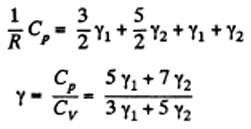13. A thermally insulated vessel with gaseous nitrogen at a temperature t = 27°C moves with velocity v = 100 m/s. How much (in per cent) and in what way will the gas pressure change on a sudden stoppage of the vessel?

Solution:

1. The internal energy of the molecules are

$U = \frac{1}{2}mN < (\vec{u} - \vec{v})^2 > = \frac{1}{2}mN $

When the vessel is stopped, internal energy becomes (1/2)mN<u2>

So, there is an increase in internal energy of ∆U = (1/2)mNv2

This will give rise to a rise in temperature of

∆T = [(1/2)mNv2]/[(i/2)R] = mNv2/iR

There being no flow of heat, this change of temperature will lead to an excess pressure

∆p = R∆T/V = mNv2/iV

And finally, ∆p/p = Mv2/iRT = 2.2%

Where M = molecular weight of N2, i = number of degrees of freedom of N2.

14. Calculate at the temperature t = 17°C:

1. (a) the root mean square velocity and the mean kinetic energy of an oxygen molecule in the process of translational motion;

2. (b) the root mean square velocity of a water droplet of diameter d = 0.10 μm suspended in the air.

Solution:

1. (a) From the equipartition theorem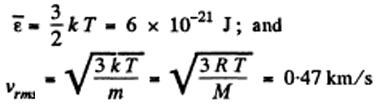(b) In equilibrium the mean kinetic energy of the droplet will be equal to that of a molecule.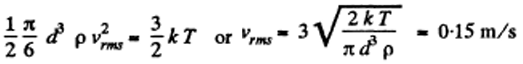15. A gas consisting of rigid diatomic molecules is expanded adiabatically. How many times has the gas to be expanded to reduce the root mean square velocity of the molecules η = 1.50 times?

Solution:

1. Here i = 5, Cv = (5/2)R and γ = 7/5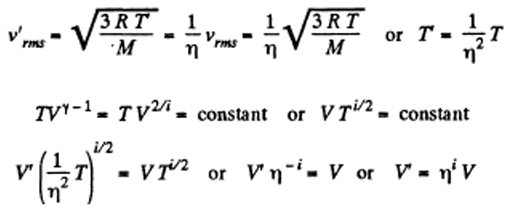The gas must be expanded ηi times i.e. 7.6 times.

16. The mass m = 15 g of nitrogen is enclosed in a vessel at a temperature T = 300 K. What amount of heat has to be transferred to the gas to increase the root mean square velocity of its molecules η = 2.0 times?

Solution:

1. Here Cv = (5/2)R m/M (i = 5)

m = mass of the gas, M = molecular weight.

If vrms increases η times, the temperature will have increased η2 times. This will require (neglecting expansion of the vessels) a heat flow of amount.

(5/2)R (m/M)( η2-1)T = 10kJ

17. The temperature of a gas consisting of rigid diatomic molecules is T = 300 K. Calculate the angular root mean square velocity of a rotating molecule if its moment of inertia is equal to I = 2.1.10-39 g cm2.

Solution:

1. The root mean square angular velocity is given by

(1/2)Iω2 = 2 x (1/2) kT (2 degree of rotations)

Or ω = √(2kT/I) = 6.3 x 1012 rad/s

18. A gas consisting of rigid diatomic molecules was initially under standard conditions. Then the gas was compressed adiabatically η = 5.0 times. Find the mean kinetic energy of a rotating molecule in the final state.

Solution:

1. Under compression, the temperature will rise

TVγ-1 = constant, TV2/i = constant

Or T’(η-1V0)2/i = ToVo2/i or T’ = η+2/iTo

19. How will the rate of collisions of rigid diatomic molecules against the vessel's wall change, if the gas is expanded adiabatically η times?

Solution:

1. Number of collision = (1/4)n<v> = v

Now, v'/v = (n'/n)(<v'>/<v> = (1/η) √(T'/T)

(When the gas is expanded η times, n decreases by a factor η).

Also, T’(ηV)2/i = TV2/i or T’ = η2/iT

So, v’/v = 1/η x η-1/i = η((-i-1)/i)

That is, collisions decrease by a factor η(i+1)/i, i = 5 here.

20. The volume of gas consisting of rigid diatomic molecules was increased η = 2.0 times in a polytrophic process with the molar heat capacity C = R. How many times will the rate of collisions of molecules against a vessel's wall be reduced as a result of this process?

Solution:

1. In a polytrophic process pVn = constant. where n is called the polytrophic index.

For this process: